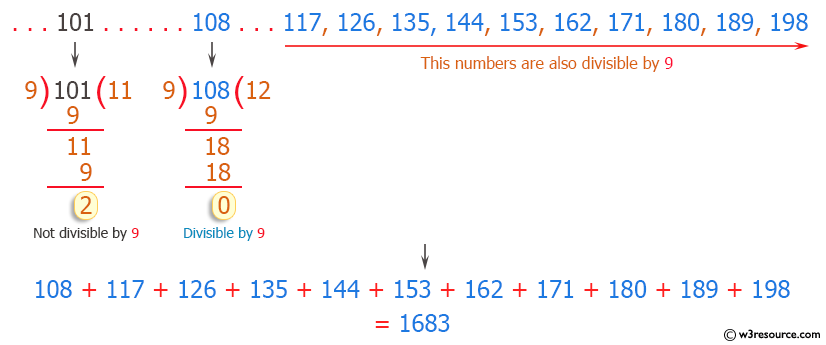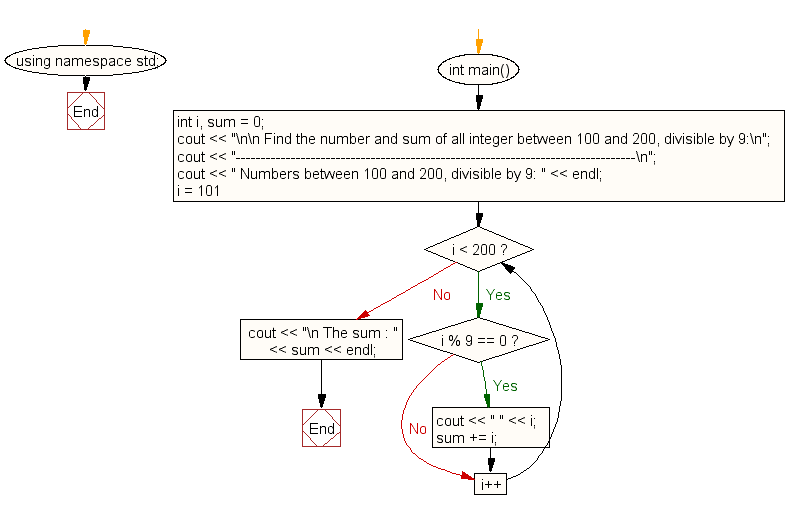﻿ C++ : Number, sum of all integer divisible by 9 in a range

# C++ Exercises: Find the number and sum of all integer between 100 and 200 which are divisible by 9

## C++ For Loop: Exercise-28 with Solution

Write a program in C++ to find the number and sum of all integers between 100 and 200 which are divisible by 9.

Pictorial Presentation:Sample Solution:-

C++ Code :

``````#include <iostream>
using namespace std;

int main()
{
int i, sum = 0;
cout << "\n\n Find the number and sum of all integer between 100 and 200, divisible by 9:\n";
cout << "--------------------------------------------------------------------------------\n";
cout << " Numbers between 100 and 200, divisible by 9: " << endl;
for (i = 101; i < 200; i++)
{
if (i % 9 == 0)
{
cout << " " << i;
sum += i;
}
}
cout << "\n The sum : " << sum << endl;
}
``````

Sample Output:

``` Find the number and sum of all integer between 100 and 200, divisible by 9:
--------------------------------------------------------------------------------
Numbers between 100 and 200, divisible by 9:
108 117 126 135 144 153 162 171 180 189 198
The sum : 1683
```

Flowchart:C++ Code Editor:

What is the difficulty level of this exercise?

﻿

## C++ Programming: Tips of the Day

What is the "-->" operator in C/C++?

--> is not an operator. It is in fact two separate operators, -- and >.

The conditional's code decrements x, while returning x's original (not decremented) value, and then compares the original value with 0 using the > operator.

To better understand, the statement could be written as follows:

while( (x--) > 0 )

Ref : https://bit.ly/3kOTBby

We are closing our Disqus commenting system for some maintenanace issues. You may write to us at reach[at]yahoo[dot]com or visit us at Facebook